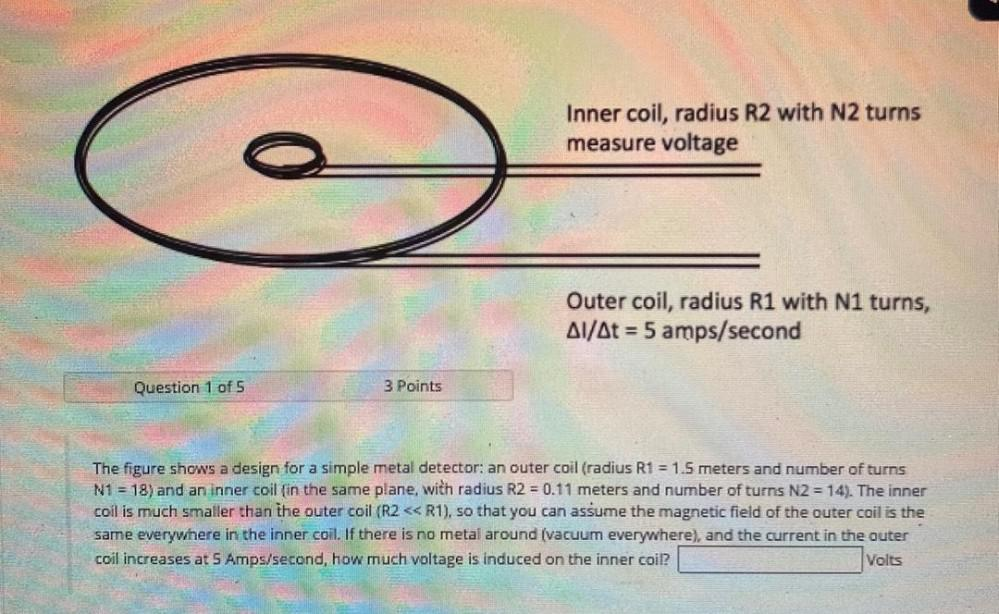Question:

# Inner coil, radius R2 with N2 turns measure voltage Outer coil, radius R1 with N1 turns, Al/At = 5 amps/second Question 1 of 5 3Inner coil, radius R2 with N2 turns measure voltage Outer coil, radius R1 with N1 turns, Al/At = 5 amps/second Question 1 of 5 3 Points The figure shows a design for a simple metal detector: an outer coil (radius R1 = 1.5 meters and number of turns N1 = 18) and an inner coil (in the same plane, with radius R2 = 0.11 meters and number of turns N2 = 14). The inner coil is much smaller than the outer coil (R2 << R1), so that you can assume the magnetic field of the outer coil is the same everywhere in the inner coil. If there is no metal around (vacuum everywhere), and the current in the outer coil increases at 5 Amps/second, how much voltage is induced on the inner coil? Volts